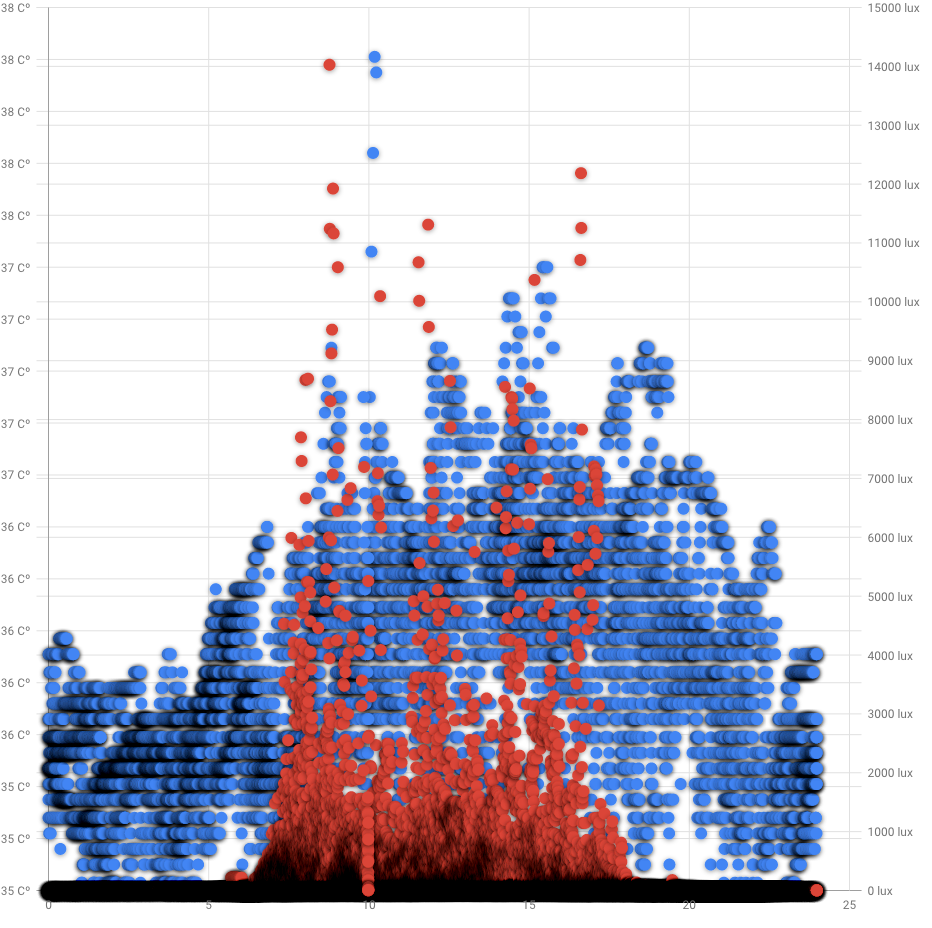Quantified Self Experiments / QS - Circadian rhythm of body temperature with iButton

## Abstract

#### What did i do?

I've measured my core body temperature circadian rhythm, to make sure it's present and is not disturbed (i've had a few years of shift work 10 years ago).

#### How did i do it?

I've used iButton placed in armpit and MetaMotionR placed at open part of wrist to measure exposure to ambient light, for 2 weeks.

#### What did i learn?

My body temperature circadian rhythm is present and looks strong enough.

## Introduction

Circadian rhythm disorders may decrease our healthspan and increase occurrence of chronic diseases during aging.

The purpose of this experiment (n=1) is to find diurnal changes in body temperature.

## Materials & Methods

#### Participants

Adult male (n=1) anthropometrics was described in previous article

#### Experimental design

From 2020-08-20 to 2020-09-04 (14 days) body temperature was measured by iButton placed inside armpit, covered by medical adhesive patch. iButton was taken out for shower / swimming activities and after finishing patch was re-applied.

Ambient light in lux was measured by MetaMotionR placed at the open part of a wrist.

## Results

Visual inspection of data reveals circadian rhythm:Blue dots is a armpit temperature in celsius  (left Y axis), red is ambient light in lux (right Y axis). X axis is a hour of a day. Google Developer charts for some reasons doesnt show decimal point for temperature and adding empty 25 hour. Measurements density highlighted by shadowing.

Day vs Night temperature

 Mean 95% CI Day (9:00 - 19:00) 35.56℃ [35.55,35.57] Night (21:00 - 7:00) 36.13℃ [36.12,36.14]

Confidence intervals a pretty narrow and far away enough to prove statistically significant difference between day and night.

Group differences

 Effect size 95% CI Hedges g Large [-1.673,-1.673] Mean difference -0.5716℃ [-0.5716, -0.5716]

We can see a pretty narrow (n~5000) CI's showing a difference of 0.57℃ between day and night with "Large" effect size.

## Discussion

The main result of that experiment is 0.57℃ difference between day and night which acts as a proxy of my circadian rhythm strength. I'll continue checking it in future, to see the trends.

## Data availability & Information

Welcome for questions, suggestions and critics in comments below.

Data is fully available here.

R Code:

`library(goftest)library(dplyr)library(effectsize)library(lubridate)library(ggplot2)library(boot)library(bootES)temp <- read.csv("https://blog.kto.to/uploads/circadian-ibutton.csv")temp <- temp[!is.na(temp\$armpittemp),]#filter 21:00 - 7:00 as group 1, 9:00 - 19:00 as group 2, other as group 0temp <- temp %>% select(datetime,armpittemp)  %>%  mutate(group = if_else(((hour(datetime) >= 21) | (hour(datetime) <=  7)), 1,                  if_else(((hour(datetime) <= 19) & (hour(datetime) >=  9)),2, 0)));#remove group 0temp <- temp[temp\$group != 0,]#check for normality distributionst <-ad.test(temp\$armpittemp) #shapiro.test for n < 5000,  ad.test otherviseif_else(st\$p.value < 0.05, 'non-uniform distribution', 'uniform distribution')bootMean <- function(data, i) { d <- data[i]; return(mean(d)); }bootMeanDiff <- function(data, i) {   d <- data;  data_group1 <- !is.na(d\$group)&d\$group == 1  data_group2 <- !is.na(d\$group)&d\$group == 2  g1 <- d\$armpittemp[data_group1]  g2 <- d\$armpittemp[data_group2]  return(mean(g1) - mean(g2)); }bootHedgesG <- function(data, i) #calc hedges G to measure effect sizes{   d <- data;  data_group1 <- !is.na(d\$group)&d\$group == 1  data_group2 <- !is.na(d\$group)&d\$group == 2  g1 <- d\$armpittemp[data_group1]  g2 <- d\$armpittemp[data_group2]  h <- hedges_g(g1, g2)  return(h\$Hedges_g); }#bootstrap hedges G effect size between group 1 and 2results <- boot(data=temp, statistic=bootHedgesG, R=10000)boot_result <- boot.ci(results, type = c("perc")); boot_result;interpret_g(1.673, "cohen1988");#bootstrap mean difference between group 1 and 2results <- boot(data=temp, statistic=bootMeanDiff, R=10000)boot_result <- boot.ci(results, type = c("perc")); boot_result;interpret_g(1.673, "cohen1988");#take out group 1 and 2 from datasetdata_group <- !is.na(temp\$group)&temp\$group == 1day <- temp\$armpittemp[data_group]data_group <- !is.na(temp\$group)&temp\$group == 2night <- temp\$armpittemp[data_group]#calc averages & CIresults <- boot(data=day, statistic=bootMean, R=10000)day_result <- boot.ci(results, type = c("perc")); day_result;results <- boot(data=night, statistic=bootMean, R=10000)night_result <- boot.ci(results, type = c("perc")); night_result;`
` "non-uniform distribution"`
`BOOTSTRAP CONFIDENCE INTERVAL CALCULATIONSBased on 10000 bootstrap replicatesCALL : boot.ci(boot.out = results, type = c("perc"))Intervals : Level     Percentile     95%   (-1.673, -1.673 )  Calculations and Intervals on Original Scale`
BOOTSTRAP CONFIDENCE INTERVAL CALCULATIONS
Based on 10000 bootstrap replicates

CALL :
boot.ci(boot.out = results, type = c("perc"))

Intervals :
Level     Percentile
95%   (35.55, 35.57 )
Calculations and Intervals on Original Scale
BOOTSTRAP CONFIDENCE INTERVAL CALCULATIONS
Based on 10000 bootstrap replicates

CALL :
boot.ci(boot.out = results, type = c("perc"))

Intervals :
Level     Percentile
95%   (36.12, 36.14 )
Calculations and Intervals on Original Scale
 "large"
`BOOTSTRAP CONFIDENCE INTERVAL CALCULATIONSBased on 10000 bootstrap replicatesCALL : boot.ci(boot.out = results, type = c("perc"))Intervals : Level     Percentile     95%   (-0.5716, -0.5716 )  Calculations and Intervals on Original Scale`
` `

#### Statistical analysis

RStudio version 1.3.959  and R version 4.0.2. Confidence Intervals was build using bootstrap. Effect sizes based on Hedge's g, Cohen's 1988 rules